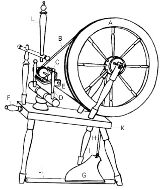# How to best implement an iterator

yourbasic.org/golangGo has a built-in range loop for iterating over slices, arrays, strings, maps and channels. See 4 basic range loop (for-each) patterns.

To iterate over other types of data, an iterator function with callbacks is a clean and fairly efficient abstraction.

## Basic iterator pattern

``````// Iterate calls the f function with n = 1, 2, and 3.
func Iterate(f func(n int)) {
for i := 1; i <= 3; i++ {
f(i)
}
}``````

In use:

``Iterate(func(n int) { fmt.Println(n) })``
``````1
2
3``````

## Iterator with break

``````// Iterate calls the f function with n = 1, 2, and 3.
// If f returns true, Iterate returns immediately
// skipping any remaining values.
func Iterate(f func(n int) (skip bool)) {
for i := 1; i <= 3; i++ {
if f(i) {
return
}
}
}``````

In use:

``````Iterate(func(n int) (skip bool) {
fmt.Println(n)
return n == 2
})``````
``````1
2``````

### More code examplesGo blueprints: code for com­mon tasks is a collection of handy code examples.